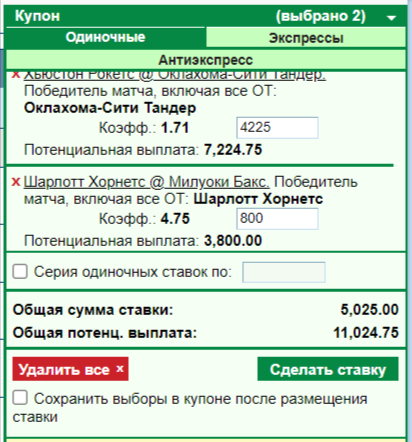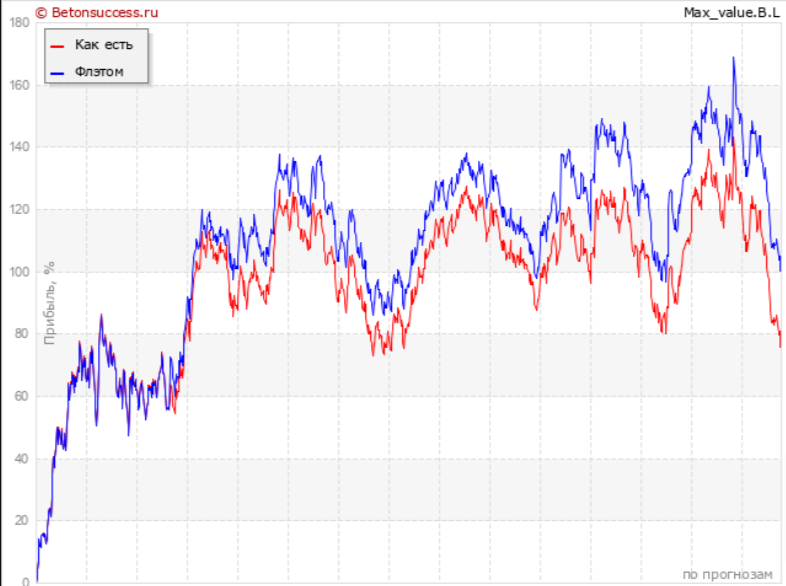# Fixed-profit betting strategy

02.12.2021

Fixed Income Strategy is one of the most adequate representatives of financial systems for bankroll management in sports betting. The strategy in its essence is very close to the traditional and American flat, but at the same time it even has a number of advantages over them. Let's figure out what it is.

## What is the difference between fixed income strategy, traditional and american flat?

All three of these systems fall into the category of even sports betting bankroll distribution strategies best suited to professional betting. The only difference is in the fixing object:

1. In a traditional flat, the amount of the bet is fixed. That is, the same amount is put on each choice, regardless of the quotes. There is also a kind of dynamic flat, in which the amount changes, but the percentage of the current bank remains unchanged.

1. In the American flat, the payout amount is fixed at the rate. That is, we bet less on high odds, and more on low odds, so that the payout from each bet is approximately the same. For example, to make payments for all bets equal to 5000 rubles.

1. In the strategy we are considering today, the net profit from the bet is fixed. That is, the net winnings minus the amount of the bet. Here, as in the American flat, there is a dependence of the size on the level of the coefficient; less money is placed on high quotes than on low ones.

## An example of betting on a fixed profit strategyTake the outcomes of two NBA basketball games. In the first game, we bet on the favorite, Oklahoma, with odds of 1.71. In the second match we believe in the underdog, “Charlotte,” and the odds are much higher. According to the fixed profit strategy, we distribute the bet amounts in such a way that the net profit from the outcome is equal.

1. 7225 - 4225 = 3000 rubles of net profit
2. 3800 - 800 = 3000 rubles of net profit

The amounts of bets differ greatly, the size of the payment also differs almost twice, but if we talk about the net profit, then it will be the same in both cases. For a quick calculation of the bet amount, there is a special simple formula.

## F / (K – 1) = S

Where F is the sum of the fixed profit (in our case 3000), which we determine at the beginning of the game using this strategy. K is the outcome rate. As a result, we get S - the amount of the bet on a specific outcome for this strategy.

We check the calculations using the formula:

1. 3000 / (1.71 - 1) = 4225 rubles
2. 3000 / (4.75 - 1) = 800 rubles

Which completely coincides with the numbers calculated in the bet coupon.

## How to calculate the optimal size of a fixed profit?

Traditionally, it is believed that the optimal size of the fixed profit in this strategy is 2-3% of the bankroll. In the presence of a large bank, it is even desirable to reduce it to 1-2% of the bank, for example, 5-10 thousand rubles with a bank of half a million. It is much safer, and the profit in money will be achieved due to the large turnover.

If the bank is very small, then you will have to risk increasing the amount of fixed profit to the maximum allowable value of 5%. If you earn 1% of a bank of 20,000 rubles (200 rubles), then the profit will grow very slowly compared to a fixed profit of 5% (1000 rubles). But after you have doubled your bankroll 2-3 times, it is highly desirable to reduce the bet amount to 2-3%.

***

In addition to other advantages of the strategy, it is still very easy to calculate the potential return on it. Only two variables appear in the calculation formula - the average permeability percentage and the amount of fixed profit. By multiplying them, you can easily get the average expected return from one bet in the money. And multiplying this amount by the estimated turnover (the number of bets) - display the amount of the average expected profitability for a month or a year.

***

## Who is the fixed profit strategy suitable for?

The strategy is perfect for beginners and those who have not yet learned how to determine the probabilities of outcomes well enough. At the very least, it will insure against unnecessary losses at non-reimbursable high coefficients. But there is one caveat - when you learn how to select really valuable high odds, then using the classic flat will become more profitable.For example, on this chart, the real profit of the bettor is regularly below the profit line that he could have taken with static flat bets. This is precisely because he is flirting with high odds for a lower percentage of the bank, and at the same time, he gets a good amount of profit from them.

In general, the fixed profit strategy is suitable for all bettors who want to play correctly. In some situations, it is even better applicable than the classic flat. For example, if a player is used to playing a very wide range of odds on a regular basis from 1.60 to 4.00 and even higher. Then the fixed profit will help him to raise money with low ratios and minimize risks on high quotes.